Normal view

# Introduction to linear regression analysis / Douglas C. Montgomery, Elizabeth A. Peck, G. Geoffrey Vining.

Material type:TextLanguage: English Series: Wiley series in probability and statistics ; 821Publication details: 2012. Edition: 5th edDescription: xvi, 645 p. : ill. ; 27 cmISBN: 9780470542811 (hardback)DDC classification: 519.536 INT LOC classification: QA278.2 | .M65 2012Other classification: MAT029000 Online resources: Cover image | OCLC | Ebook Fulltext
Contents:
PREFACE xiii 1. INTRODUCTION 1 1.1 Regression and Model Building 1 1.2 Data Collection 5 1.3 Uses of Regression 9 1.4 Role of the Computer 10 2. SIMPLE LINEAR REGRESSION 12 2.1 Simple Linear Regression Model 12 2.2 Least-Squares Estimation of the Parameters 13 2.3 Hypothesis Testing on the Slope and Intercept 22 2.4 Interval Estimation in Simple Linear Regression 29 2.5 Prediction of New Observations 33 2.6 Coeffi cient of Determination 35 2.7 A Service Industry Application of Regression 37 2.8 Using SAS and R for Simple Linear Regression 39 2.9 Some Considerations in the Use of Regression 42 2.10 Regression Through the Origin 45 2.11 Estimation by Maximum Likelihood 51 2.12 Case Where the Regressor x is Random 52 3. MULTIPLE LINEAR REGRESSION 67 3.1 Multiple Regression Models 67 3.2 Estimation of the Model Parameters 70 3.3 Hypothesis Testing in Multiple Linear Regression 84 3.4 Confidence Intervals in Multiple Regression 97 3.5 Prediction of New Observations 104 3.6 A Multiple Regression Model for the Patient Satisfaction Data 104 3.7 Using SAS and R for Basic Multiple Linear Regression 106 3.8 Hidden Extrapolation in Multiple Regression 107 3.9 Standardized Regression Coeffi cients 111 3.10 Multicollinearity 117 3.11 Why Do Regression Coeffi cients Have the Wrong Sign? 119 4. MODEL ADEQUACY CHECKING 129 4.1 Introduction 129 4.2 Residual Analysis 130 4.3 PRESS Statistic 151 4.4 Detection and Treatment of Outliers 152 4.5 Lack of Fit of the Regression Model 156 5. TRANSFORMATIONS AND WEIGHTING TO CORRECT MODEL INADEQUACIES 171 5.1 Introduction 171 5.2 Variance-Stabilizing Transformations 172 5.3 Transformations to Linearize the Model 176 5.4 Analytical Methods for Selecting a Transformation 182 5.5 Generalized and Weighted Least Squares 188 5.6 Regression Models with Random Effect 194 6. DIAGNOSTICS FOR LEVERAGE AND INFLUENCE 211 6.1 Importance of Detecting Infl uential Observations 211 6.2 Leverage 212 6.3 Measures of Infl uence: Cook's D 215 6.4 Measures of Infl uence: DFFITS and DFBETAS 217 6.5 A Measure of Model Performance 219 6.6 Detecting Groups of Infl uential Observations 220 6.7 Treatment of Infl uential Observations 220 7. POLYNOMIAL REGRESSION MODELS 223 7.1 Introduction 223 7.2 Polynomial Models in One Variable 223 7.3 Nonparametric Regression 236 7.4 Polynomial Models in Two or More Variables 242 7.5 Orthogonal Polynomials 248 8. INDICATOR VARIABLES 260 8.1 General Concept of Indicator Variables 260 8.2 Comments on the Use of Indicator Variables 273 8.3 Regression Approach to Analysis of Variance 275 9. MULTICOLLINEARITY 285 9.1 Introduction 285 9.2 Sources of Multicollinearity 286 9.3 Effects of Multicollinearity 288 9.4 Multicollinearity Diagnostics 292 9.5 Methods for Dealing with Multicollinearity 303 9.6 Using SAS to Perform Ridge and Principal-Component Regression 321 10. VARIABLE SELECTION AND MODEL BUILDING 327 10.1 Introduction 327 10.2 Computational Techniques for Variable Selection 338 10.3 Strategy for Variable Selection and Model Building 351 10.4 Case Study: Gorman and Toman Asphalt Data Using SAS 354 11. VALIDATION OF REGRESSION MODELS 372 11.1 Introduction 372 11.2 Validation Techniques 373 11.3 Data from Planned Experiments 385 12. INTRODUCTION TO NONLINEAR REGRESSION 389 12.1 Linear and Nonlinear Regression Models 389 12.2 Origins of Nonlinear Models 391 12.3 Nonlinear Least Squares 395 12.4 Transformation to a Linear Model 397 12.5 Parameter Estimation in a Nonlinear System 400 12.6 Statistical Inference in Nonlinear Regression 409 12.7 Examples of Nonlinear Regression Models 411 12.8 Using SAS and R 412 13. GENERALIZED LINEAR MODELS 421 13.1 Introduction 421 13.2 Logistic Regression Models 422 13.3 Poisson Regression 444 13.4 The Generalized Linear Model 450 14. REGRESSION ANALYSIS OF TIME SERIES DATA 474 14.1 Introduction to Regression Models for Time Series Data 474 14.2 Detecting Autocorrelation: The Durbin-Watson Test 475 14.3 Estimating the Parameters in Time Series Regression Models 480 15. OTHER TOPICS IN THE USE OF REGRESSION ANALYSIS 500 15.1 Robust Regression 500 15.2 Effect of Measurement Errors in the Regressors 511 15.3 Inverse Estimation--The Calibration Problem 513 15.4 Bootstrapping in Regression 517 15.5 Classifi cation and Regression Trees (CART) 524 15.6 Neural Networks 526 15.7 Designed Experiments for Regression 529 APPENDIX A. STATISTICAL TABLES 541 APPENDIX B. DATA SETS FOR EXERCISES 553 APPENDIX C. SUPPLEMENTAL TECHNICAL MATERIAL 574 C.1 Background on Basic Test Statistics 574 C.2 Background from the Theory of Linear Models 577 C.3 Important Results on SSR and SSRes 581 C.4 Gauss-Markov Theorem, Var(epsilon) = sigma2I 587 C.5 Computational Aspects of Multiple Regression 589 C.6 Result on the Inverse of a Matrix 590 C.7 Development of the PRESS Statistic 591 C.8 Development of S2 (i) 593 C.9 Outlier Test Based on R-Student 594 C.10 Independence of Residuals and Fitted Values 596 C.11 Gauss--Markov Theorem, Var(epsilon) = V 597 C.12 Bias in MSRes When the Model Is Underspecifi ed 599 C.13 Computation of Infl uence Diagnostics 600 C.14 Generalized Linear Models 601 APPENDIX D. INTRODUCTION TO SAS 613 D.1 Basic Data Entry 614 D.2 Creating Permanent SAS Data Sets 618 D.3 Importing Data from an EXCEL File 619 D.4 Output Command 620 D.5 Log File 620 D.6 Adding Variables to an Existing SAS Data Set 622 APPENDIX E. INTRODUCTION TO R TO PERFORM LINEAR REGRESSION ANALYSIS 623 E.1 Basic Background on R 623 E.2 Basic Data Entry 624 E.3 Brief Comments on Other Functionality in R 626 E.4 R Commander 627 REFERENCES 628 INDEX 642
Summary: "This book describes both the conventional and less common uses of linear regression in the practical context of today's mathematical and scientific research"--
Tags from this library: No tags from this library for this title.
Star ratingsAverage rating: 0.0 (0 votes)
Holdings
Item type Current library Collection Call number Copy number Status Date due Barcode Item holdsE-Book
E-book
Non-fiction 519.536 INT 2012 (Browse shelf(Opens below)) Not for loanText
Reserve Section
Non-fiction 519.536 INT 2012 (Browse shelf(Opens below)) C-1 Not For Loan 25374
Total holds: 0
##### Browsing EWU Library shelves, Shelving location: Reserve Section Close shelf browser (Hides shelf browser)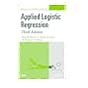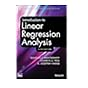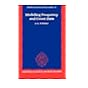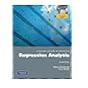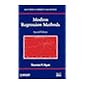519.536 HOA 2013 Applied logistic regression. 519.536 HOA 2013 Applied logistic regression. 519.536 HOA 2013 Applied logistic regression / 519.536 INT 2012 Introduction to linear regression analysis / 519.536 LIM 1995 Modelling frequency and count data / 519.536 MES 2012 A second course in statistics : 519.536 RYM 2009 Modern regression methods /

Includes bibliographical references (p. 628-641) and index.

PREFACE xiii 1. INTRODUCTION 1 1.1 Regression and Model Building 1 1.2 Data Collection 5 1.3 Uses of Regression 9 1.4 Role of the Computer 10 2. SIMPLE LINEAR REGRESSION 12 2.1 Simple Linear Regression Model 12 2.2 Least-Squares Estimation of the Parameters 13 2.3 Hypothesis Testing on the Slope and Intercept 22 2.4 Interval Estimation in Simple Linear Regression 29 2.5 Prediction of New Observations 33 2.6 Coeffi cient of Determination 35 2.7 A Service Industry Application of Regression 37 2.8 Using SAS and R for Simple Linear Regression 39 2.9 Some Considerations in the Use of Regression 42 2.10 Regression Through the Origin 45 2.11 Estimation by Maximum Likelihood 51 2.12 Case Where the Regressor x is Random 52 3. MULTIPLE LINEAR REGRESSION 67 3.1 Multiple Regression Models 67 3.2 Estimation of the Model Parameters 70 3.3 Hypothesis Testing in Multiple Linear Regression 84 3.4 Confidence Intervals in Multiple Regression 97 3.5 Prediction of New Observations 104 3.6 A Multiple Regression Model for the Patient Satisfaction Data 104 3.7 Using SAS and R for Basic Multiple Linear Regression 106 3.8 Hidden Extrapolation in Multiple Regression 107 3.9 Standardized Regression Coeffi cients 111 3.10 Multicollinearity 117 3.11 Why Do Regression Coeffi cients Have the Wrong Sign? 119 4. MODEL ADEQUACY CHECKING 129 4.1 Introduction 129 4.2 Residual Analysis 130 4.3 PRESS Statistic 151 4.4 Detection and Treatment of Outliers 152 4.5 Lack of Fit of the Regression Model 156 5. TRANSFORMATIONS AND WEIGHTING TO CORRECT MODEL INADEQUACIES 171 5.1 Introduction 171 5.2 Variance-Stabilizing Transformations 172 5.3 Transformations to Linearize the Model 176 5.4 Analytical Methods for Selecting a Transformation 182 5.5 Generalized and Weighted Least Squares 188 5.6 Regression Models with Random Effect 194 6. DIAGNOSTICS FOR LEVERAGE AND INFLUENCE 211 6.1 Importance of Detecting Infl uential Observations 211 6.2 Leverage 212 6.3 Measures of Infl uence: Cook's D 215 6.4 Measures of Infl uence: DFFITS and DFBETAS 217 6.5 A Measure of Model Performance 219 6.6 Detecting Groups of Infl uential Observations 220 6.7 Treatment of Infl uential Observations 220 7. POLYNOMIAL REGRESSION MODELS 223 7.1 Introduction 223 7.2 Polynomial Models in One Variable 223 7.3 Nonparametric Regression 236 7.4 Polynomial Models in Two or More Variables 242 7.5 Orthogonal Polynomials 248 8. INDICATOR VARIABLES 260 8.1 General Concept of Indicator Variables 260 8.2 Comments on the Use of Indicator Variables 273 8.3 Regression Approach to Analysis of Variance 275 9. MULTICOLLINEARITY 285 9.1 Introduction 285 9.2 Sources of Multicollinearity 286 9.3 Effects of Multicollinearity 288 9.4 Multicollinearity Diagnostics 292 9.5 Methods for Dealing with Multicollinearity 303 9.6 Using SAS to Perform Ridge and Principal-Component Regression 321 10. VARIABLE SELECTION AND MODEL BUILDING 327 10.1 Introduction 327 10.2 Computational Techniques for Variable Selection 338 10.3 Strategy for Variable Selection and Model Building 351 10.4 Case Study: Gorman and Toman Asphalt Data Using SAS 354 11. VALIDATION OF REGRESSION MODELS 372 11.1 Introduction 372 11.2 Validation Techniques 373 11.3 Data from Planned Experiments 385 12. INTRODUCTION TO NONLINEAR REGRESSION 389 12.1 Linear and Nonlinear Regression Models 389 12.2 Origins of Nonlinear Models 391 12.3 Nonlinear Least Squares 395 12.4 Transformation to a Linear Model 397 12.5 Parameter Estimation in a Nonlinear System 400 12.6 Statistical Inference in Nonlinear Regression 409 12.7 Examples of Nonlinear Regression Models 411 12.8 Using SAS and R 412 13. GENERALIZED LINEAR MODELS 421 13.1 Introduction 421 13.2 Logistic Regression Models 422 13.3 Poisson Regression 444 13.4 The Generalized Linear Model 450 14. REGRESSION ANALYSIS OF TIME SERIES DATA 474 14.1 Introduction to Regression Models for Time Series Data 474 14.2 Detecting Autocorrelation: The Durbin-Watson Test 475 14.3 Estimating the Parameters in Time Series Regression Models 480 15. OTHER TOPICS IN THE USE OF REGRESSION ANALYSIS 500 15.1 Robust Regression 500 15.2 Effect of Measurement Errors in the Regressors 511 15.3 Inverse Estimation--The Calibration Problem 513 15.4 Bootstrapping in Regression 517 15.5 Classifi cation and Regression Trees (CART) 524 15.6 Neural Networks 526 15.7 Designed Experiments for Regression 529 APPENDIX A. STATISTICAL TABLES 541 APPENDIX B. DATA SETS FOR EXERCISES 553 APPENDIX C. SUPPLEMENTAL TECHNICAL MATERIAL 574 C.1 Background on Basic Test Statistics 574 C.2 Background from the Theory of Linear Models 577 C.3 Important Results on SSR and SSRes 581 C.4 Gauss-Markov Theorem, Var(epsilon) = sigma2I 587 C.5 Computational Aspects of Multiple Regression 589 C.6 Result on the Inverse of a Matrix 590 C.7 Development of the PRESS Statistic 591 C.8 Development of S2 (i) 593 C.9 Outlier Test Based on R-Student 594 C.10 Independence of Residuals and Fitted Values 596 C.11 Gauss--Markov Theorem, Var(epsilon) = V 597 C.12 Bias in MSRes When the Model Is Underspecifi ed 599 C.13 Computation of Infl uence Diagnostics 600 C.14 Generalized Linear Models 601 APPENDIX D. INTRODUCTION TO SAS 613 D.1 Basic Data Entry 614 D.2 Creating Permanent SAS Data Sets 618 D.3 Importing Data from an EXCEL File 619 D.4 Output Command 620 D.5 Log File 620 D.6 Adding Variables to an Existing SAS Data Set 622 APPENDIX E. INTRODUCTION TO R TO PERFORM LINEAR REGRESSION ANALYSIS 623 E.1 Basic Background on R 623 E.2 Basic Data Entry 624 E.3 Brief Comments on Other Functionality in R 626 E.4 R Commander 627 REFERENCES 628 INDEX 642

"This book describes both the conventional and less common uses of linear regression in the practical context of today's mathematical and scientific research"--

AS

Tahur Ahmed

There are no comments on this title.